#### You may also like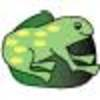### The Lily Pond

Freddie Frog visits as many of the leaves as he can on the way to see Sammy Snail but only visits each lily leaf once. Which is the best way for him to go?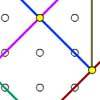### Coordinate Tan

What are the coordinates of the coloured dots that mark out the tangram? Try changing the position of the origin. What happens to the coordinates now?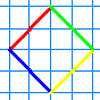### A Cartesian Puzzle

Find the missing coordinates which will form these eight quadrilaterals. These coordinates themselves will then form a shape with rotational and line symmetry.

# Fred the Class Robot

## Fred the Class Robot

Billy's school has a paved area at the front: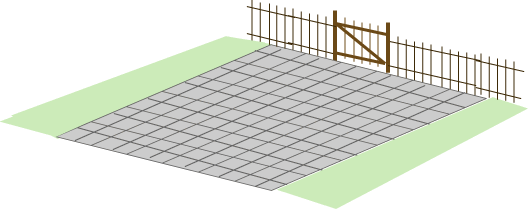Billy's class had a robot called Fred who could draw with chalk held underneath him.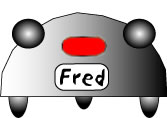Billy's teacher drew lines and numbers on the paved area in front of the school so that they could tell Fred where to go.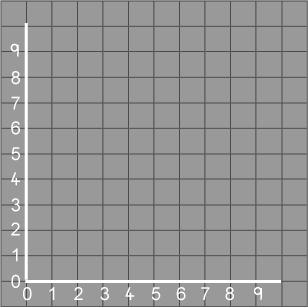Billy's teacher put a little cross on the point $(1, 2)$ and the children put Fred on the cross. Fred moved to $(4, 7)$, then to $(4, 2)$ and then back to $(1, 2 )$. Fred's chalk drew a line to show where he had been.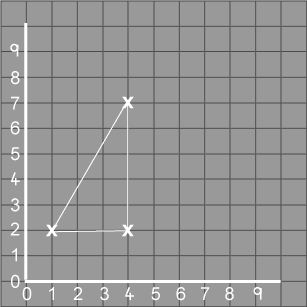"What shape has Fred drawn?" asked Billy's teacher.
"A triangle!" shouted all the class.
All the children wanted to make Fred draw shapes.
Tom had the first turn. He got Fred to go from $(6, 1)$ to $(6, 6)$, and then to $(1, 6)$ and $(1, 1)$ and back to $(6, 1)$.
Vicky made Fred go from $(2, 1)$ to $(7, 2)$, and then to $(9, 4)$ and $(4, 6)$ and then $(1, 5)$ and back to $(2, 1)$.
Billy thought very hard when it was his turn. He made Fred go from $(7, 4)$ to $(4, 7)$, and then to $(1, 4)$ and $(4, 1)$ and back to $(7, 4)$.
If Billy had made Fred start at the same place, $(7, 4)$, how else could a shape like this be drawn on the $9$ by $9$ axes? (It doesn't have to be the same size as Billy's first shape.)

### Why do this problem?

This problem is one in which learners will be practising the use of coordinates and in addition will be developing their understanding of properties of shapes. The problem may challenge their preconceptions of how a shape "should be" oriented!

A similar activity could be set up practically in the classroom or outside using a floor turtle. In any case squared paper should be provided.

### Key questions

Would it help to draw out what Fred is doing on squared paper?

Have you counted the number of sides of each shape and looked at the angles?

What makes the shape a square and not another four-sided shape?

### Possible extension

Learners could make their own shapes on squared paper and write down the coordinates.

### Possible support

Suggest drawing the shapes on squared paper. Moving the paper around might help in seeing what each shape is.